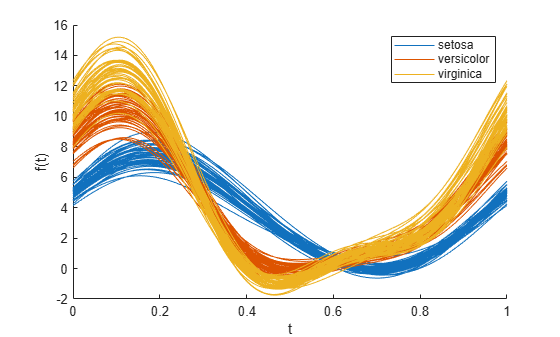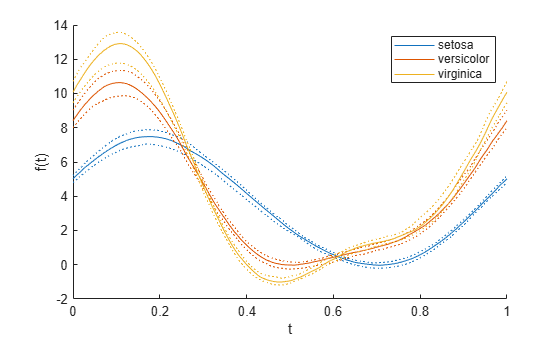# andrewsplot

## Syntax

```andrewsplot(X) andrewsplot(X,...,'Standardize',standopt) andrewsplot(X,...,'Quantile',alpha) andrewsplot(X,...,'Group',group) andrewsplot(X,...,'PropName',PropVal,...) andrewsplot(ax,X,...) h = andrewsplot(X,...) ```

## Description

`andrewsplot(X)` creates an Andrews plot of the multivariate data in the matrix `X`. The rows of `X` correspond to observations, the columns to variables. Andrews plots represent each observation by a function f(t) of a continuous dummy variable t over the interval [0,1]. f(t) is defined for the ith observation in `X` as

`$f\left(t\right)=X\left(i,1\right)/\sqrt{2}+X\left(i,2\right)\mathrm{sin}\left(2\pi t\right)+X\left(i,3\right)\mathrm{cos}\left(2\pi t\right)+\dots$`

`andrewsplot` treats `NaN` values in `X` as missing values and ignores the corresponding rows.

`andrewsplot(X,...,'Standardize',standopt)` creates an Andrews plot where `standopt` is one of the following:

• `'on'` — scales each column of `X` to have mean `0` and standard deviation `1` before making the plot.

• `'PCA'` — creates an Andrews plot from the principal component scores of `X`, in order of decreasing eigenvalue. (See `pca`.)

• `'PCAStd'` — creates an Andrews plot using the standardized principal component scores. (See `pca`.)

`andrewsplot(X,...,'Quantile',alpha)` plots only the median and the `alpha` and (1 – `alpha`) quantiles of f(t) at each value of t. This is useful if `X` contains many observations.

`andrewsplot(X,...,'Group',group)` plots the data in different groups with different colors. Groups are defined by `group`, a numeric array containing a group index for each observation. `group` can also be a categorical array, character matrix, string array, or cell array of character vectors containing a group name for each observation.

`andrewsplot(X,...,'PropName',PropVal,...)` sets optional `Line` object properties to the specified values for all `Line` objects created by `andrewsplot`. (See Line Properties.)

`andrewsplot(ax,X,...)` uses the plot axes specified in `ax`, an `Axes` object. (See `axes`.) Specify `ax` as the first input argument followed by any of the input argument combinations in the previous syntaxes.

`h = andrewsplot(X,...)` returns a column vector of handles to the `Line` objects created by `andrewsplot`, one handle per row of `X`. If you use the `'Quantile'` input parameter, `h` contains one handle for each of the three `Line` objects created. If you use both the `'Quantile'` and the `'Group'` input parameters, `h` contains three handles for each group.

## Examples

collapse all

This example shows how to create an Andrews plot to visualize grouped sample data.

`load fisheriris`

Create an Andrews plot, grouping the sample data by `species`.

`andrewsplot(meas,'group',species)`Create a second, simplified Andrews plot that only displays the median and quartiles of each group.

`andrewsplot(meas,'group',species,'quantile',.25)`## Version History

Introduced before R2006a